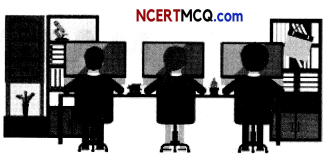Students can access the CBSE Sample Papers for Class 12 Maths with Solutions and marking scheme Term 2 Set 10 will help students in understanding the difficulty level of the exam.

## CBSE Sample Papers for Class 12 Maths Term 2 Set 10 for Practice

Time Allowed: 2 Hours
Maximum Marks: 40

General Instructions:

• This question paper contains three sections-A. B and C. Each part is compulsory.
• Section-A has 6 short answer type (SA1) questions of 2 marks each.
• Section-B has 4 short answer type (SA2) questions of 3 marks each.
• Section-C has 4 long answer type questions (LA) of 4 marks each.
• There is an Internal choice in some of the questions.
• Q14 is a case-based problem having 2 sub parts of 2 marks each.

Section – A
(Section – A has 6 short answer type (SA-1) questions of 2 marks each.)

Question 1.
Sketch the region bounded by the curve y = x2 + 2 and the lines y = x, x = 0 and x = 3. (2)

Question 2.
A bag contains 8 red and 5 white balls. Two successive draws of 3 balls are made without replacement. Find the probability that the first draw will give 3 white balls and the second draw will give 3 red balls.
OR
Three dice are thrown at the same time. Find the probability of getting three 2’s, if it is known that the sum of numbers on the three dice is six. (2)

Question 3.
Evaluate ∫$$\frac{\cos 2 x-\cos 2 \alpha}{\cos x-\cos \alpha}$$dx. (2)Question 4.
Find the coordinates of the point where the line $$\frac{x-2}{3}=\frac{y+1}{4}=\frac{z-2}{2}$$ intersect the plane x – y + z – 5 = 0. (2)

Question 5.
For any two vectors $$\vec{a}$$ and $$\vec{b}$$ , show that $$|\vec{a}+\vec{b}|^{2}+|\vec{a}-\vec{b}|^{2}=2\left(|\vec{a}|^{2}+|\vec{b}|^{2}\right)$$. (2)

Question 6.
Purse I contains 2 silver and 4 gold coins. Purse II contains 4 silver and 3 gold coins. A coin is taken out at random from one of the two purses. What is the probability that it is a silver coin? (2)

Section – B
(Section – B has 4 short answer type (SA-2) questions of 3 marks each.)

Question 7.
Evaluate ∫$$\frac{1}{(x+1)\left(x^{2}+2 x+2\right)}$$ dx
OR
Evaluate ∫-12f(x)dx, where 1(x) = |x + 1| + |x| + |x – 1|. (3)

Question 8.
Find the equation of the Line through (-1, 3, 7) and perpendicular to the lines $$\vec{r}$$ =(2î – 3ĵ) + λ(2î – 3ĵ + k̂) and $$\vec{r}$$ = (î – ĵ + k̂) + μ(7ĵ – 5k̂) (3)

Question 9.
If with reference to the right handed system of mutually perpendicular unit vectors î, ĵ, and k̂,$$\vec{α}$$ = 3î – ĵ and $$\vec{β}$$ = 2î + ĵ – 3k̂,then express in the form $$\vec{\beta}=\overrightarrow{\beta_{1}}+\overrightarrow{\beta_{2}}$$ where $$\overrightarrow{\beta_{1}}$$ is parallel to $$\vec{α}$$ and $$\overrightarrow{\beta_{2}}$$ is perpendicular to $$\vec{α}$$.
OR
Let $$\vec{a}$$ = î + 4ĵ + 2k̂, $$\vec{b}$$ = 3î – 2ĵ + 7k̂ and $$\vec{c}$$ = 2î – ĵ + 4k̂. Find a vector $$\vec{d}$$. which is perpendicular to both $$\vec{a}$$ and $$\vec{b}$$ and $$\vec{c} \cdot \vec{d}$$ = 15. (3)

Question 10.
Find the area of the region bounded by the curves y = x2 and y = |x|. (3)

Section – C
(Section – C has 4 Long answer type questions (LA) of 4 marks each.)

Question 11.
Show that the Lines $$\frac{x+1}{-3}=\frac{y-3}{2}=\frac{z+2}{1}$$ and $$\frac{x}{1}=\frac{y-7}{-3}=\frac{z+7}{2}$$ intersect. Also,find the coordinates of the point of intersection. (4)

Question 12.
Solve: x$$\frac{d y}{d x}$$ – y = $$\frac{x+1}{e^{x}}$$
OR
Find the particular solution of the differential equation $$\frac{d y}{d x}$$ = 3y cot x = sin 2x, given that y = 2 when x = $$\frac{\pi}{2}$$Question 13.
Evaluate ∫0π$$\frac{x}{a^{2} \cos ^{2} x+b^{2} \sin ^{2} x}$$dx.

Case-Based/Data-Based

Question 14.
In an office, three employees Pradeep, Pujo, and Praveen process the copies of a certain form. Pradeep process 50% of the forms Puja process 20% and Praveen processes the remaining 30% of the forms. Pradeep has an error rate of 0.06, Puja has an error rate of 0.04 and Praveen has an error rate of 0.03.Based on the above information, answer the following two questions:
(A) Find the total probability of committing an error in processing the form. (2)
(B) The manager of the office wants to do a quality check. During the inspection he selects a form at random from the day’s output of processed forms. If the form selected at random has an error, find the probability that the form is not processed by Pradeep. (2)